# Rules of exponents

By Martin McBride, 2023-02-10
Tags: exponential exponent power root
Categories: exponentialsHere is a video:

## What are exponential functions

An exponential function in x takes the form a raised to the power x, that is $a^x$. a is called the base, and x is called the exponent.

Here are the first few powers of a: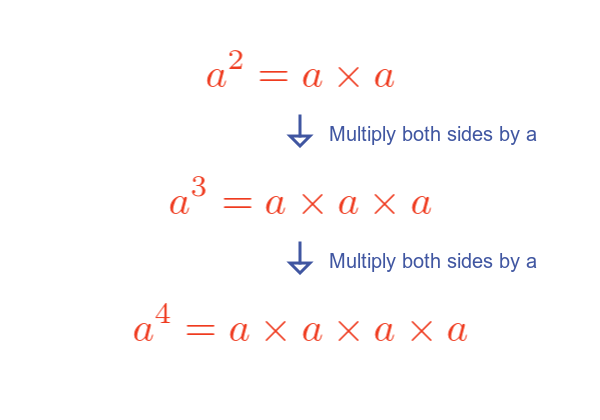$a^2$, of course, is commonly called a squared and is calculated as a multiplied by a.

$a^3$ is commonly called a cubed and is calculated as a multiplied by a multiplied by a.

$a^4$ doesn't have a special name, it can be called the fourth power of a, or a to the power four.

## Simple definition of the exponential function

Generally, $a^n$, where n is an integer of 2 or greater is the nth power of a and is often described as a multiplied by itself n times.

That isn't quite true though. a squared is actually a multiplied by itself once, not twice.

A better definition would be that $a^n$ is one multiplied by a, n times.

## Exponential values

If we chose a base of 2, we can calculate some values: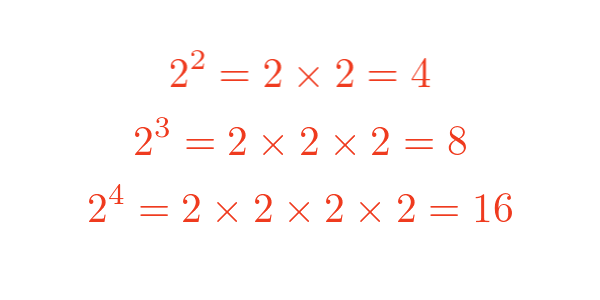As another example, if we chose a base of 10, we can get different values: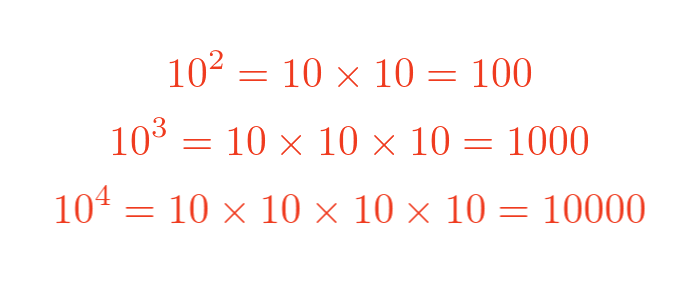## Laws of exponents

There are several laws that can be used to simplify expressions involving exponents:

• Product of powers rule.
• Quotient of powers rule.
• Power of a power rule.
• Power of a product rule.
• Power of a quotient rule.

## Product of powers rule

When multiplying two exponential terms that have the same base, we add the exponents:

$$a^p a^q = a^{p + q}$$

Here is an illustration for $a^3$ multiplied by $a^2$: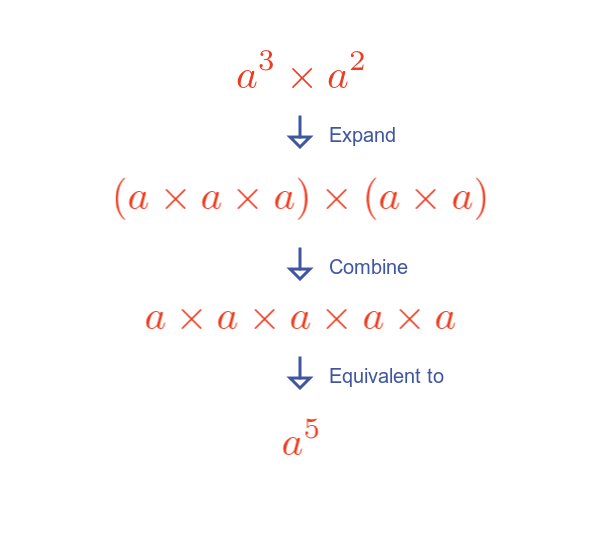Expanding each term shows that the total number of terms is 5, the sum of the exponents.

## Quotient of powers rule

When dividing two exponential terms that have the same base, subtract the exponent of the denominator from the exponent of the numerator:

$$\frac{a^p}{a^q} = a^{p - q}$$

Here is an illustration for $a^5$ divided by $a^3$: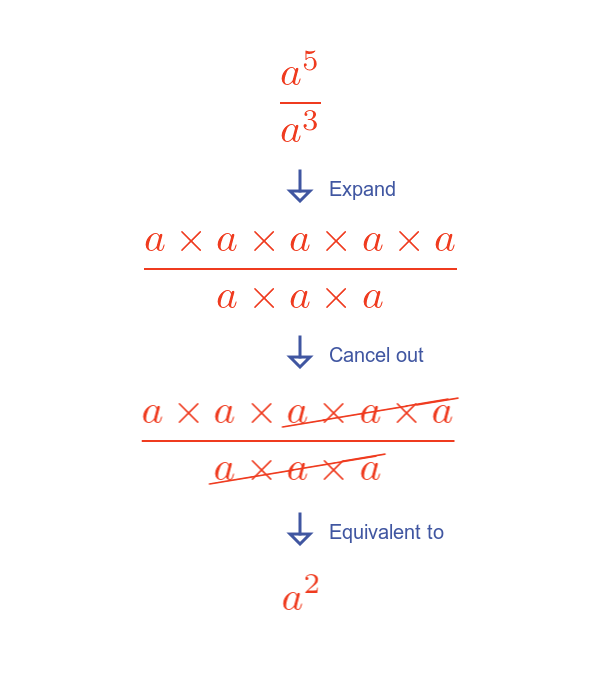Expanding each term and cancelling top and bottom shows that the total number of terms is 2, the difference between the exponents.

## Power of a power rule

When raising a power to a power, multiply the two exponents:

$${a^p}^q = a^{p q}$$

Here is an illustration for $a^3$ squared: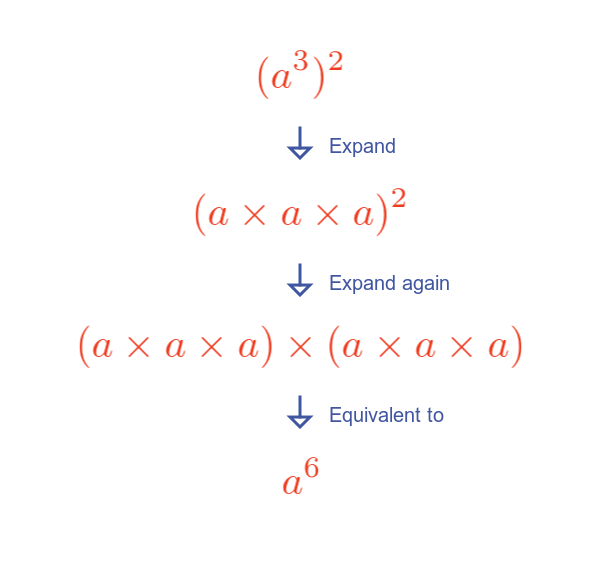Expanding each term and cancelling top and bottom shows that the total number of terms is 6, the product of the exponents.

## Power of a product rule

If we have a base that consists of a product of two (or more) terms, all raised to a power, we can simplify it by distributing the exponent to each term in the base:

$$(a b)^p = a^p b^p$$

Here is an example of ab cubed: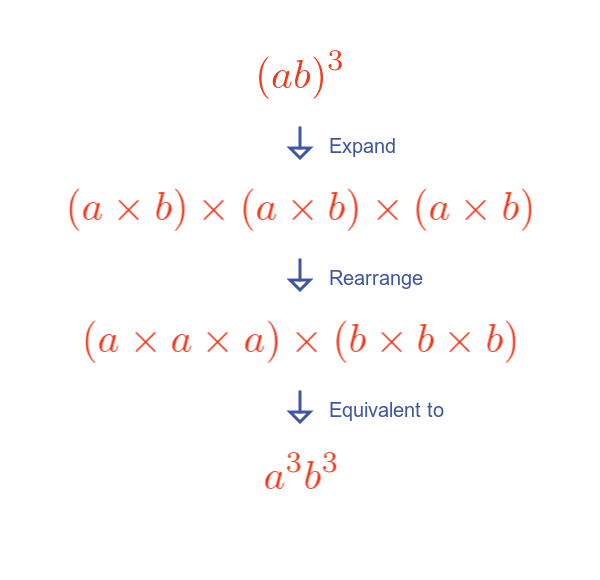Expanding and rearranging the equation for ab cubed demonstrates the rule. This can easily be extended to other powers.

## Power of a quotient rule

The previous rule also applies to the quotient of two values, all raised to a power. Again we can simplify it by distributing the power to each term in the quotient:

$$(\frac{a}{b})^p = \frac{a^p}{b^p}$$

Here is an example of a/b cubed: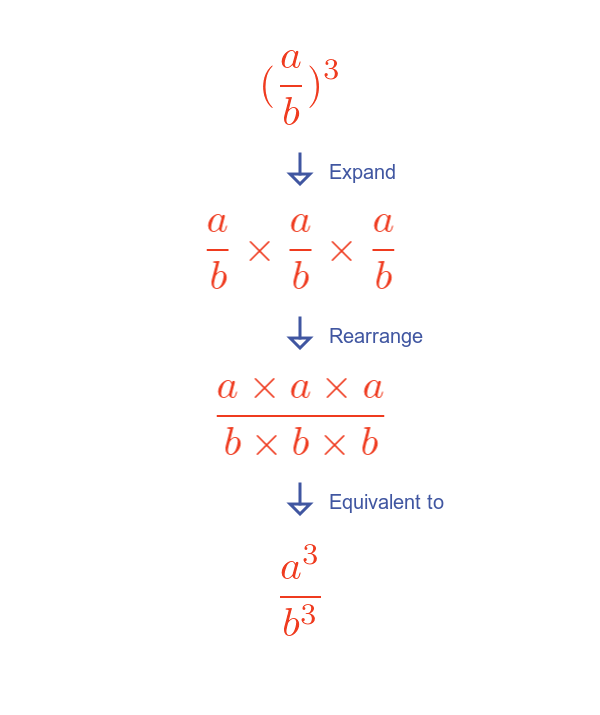Expanding and rearranging the equation for a/b cubed demonstrates the rule. This can easily be extended to other powers.